# JP2013126026A - Non-target sound suppression device, non-target sound suppression method and non-target sound suppression program - Google Patents

Non-target sound suppression device, non-target sound suppression method and non-target sound suppression program Download PDF

## Info

Publication number
JP2013126026A
JP2013126026A JP2011272618A JP2011272618A JP2013126026A JP 2013126026 A JP2013126026 A JP 2013126026A JP 2011272618 A JP2011272618 A JP 2011272618A JP 2011272618 A JP2011272618 A JP 2011272618A JP 2013126026 A JP2013126026 A JP 2013126026A
Authority
JP
Japan
Prior art keywords
coherence
target sound
means
value
Prior art date
Legal status (The legal status is an assumption and is not a legal conclusion. Google has not performed a legal analysis and makes no representation as to the accuracy of the status listed.)
Granted
Application number
JP2011272618A
Other languages
Japanese (ja)
Other versions
JP5927887B2 (en
Inventor
Katsuyuki Takahashi

Original Assignee
Oki Electric Ind Co Ltd

Priority date (The priority date is an assumption and is not a legal conclusion. Google has not performed a legal analysis and makes no representation as to the accuracy of the date listed.)
Filing date
Publication date
Application filed by Oki Electric Ind Co Ltd, 沖電気工業株式会社 filed Critical Oki Electric Ind Co Ltd
Priority to JP2011272618A priority Critical patent/JP5927887B2/en
Publication of JP2013126026A publication Critical patent/JP2013126026A/en
Application granted granted Critical
Publication of JP5927887B2 publication Critical patent/JP5927887B2/en
Application status is Active legal-status Critical
Anticipated expiration legal-status Critical

• 230000001629 suppression Effects 0 abstract title 5
• 230000015556 catabolic process Effects 0 abstract 1
• 230000004059 degradation Effects 0 abstract 1
• 238000006731 degradation Methods 0 abstract 1

## Images

•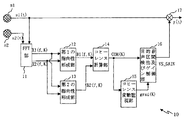••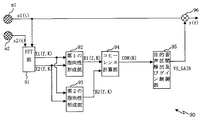••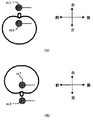•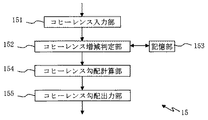•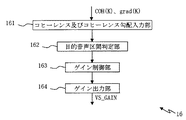•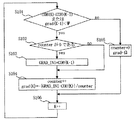•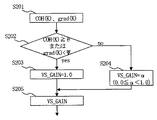•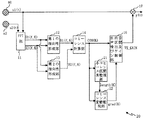•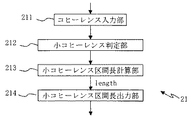•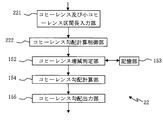•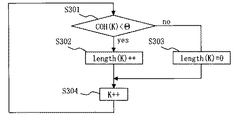•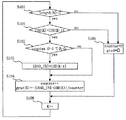•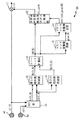•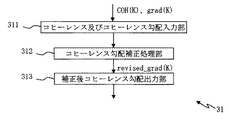••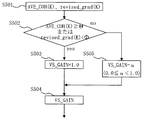•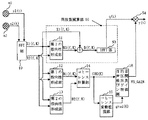•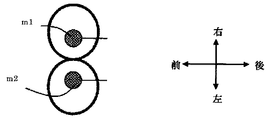•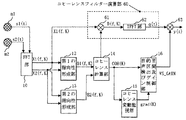•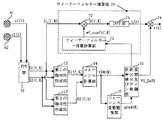## Abstract

[PROBLEMS] To accurately detect a target speech including a small amplitude component of a target speech that has been erroneously determined to be a non-target speech segment and is suppressed in spite of being a target speech segment in the prior art. By suppressing the sound component, it is possible to prevent the deterioration of sound quality that has occurred in the prior art.
The present invention converts an input signal from a time domain to a frequency domain, and performs coherence based on a signal having a first directivity and a signal having a second directivity each having a blind spot in a predetermined direction. A value is obtained, and a coherence gradient is obtained based on the coherence value. The target sound section detection means determines that the target sound section is a coherence value greater than a predetermined target sound section determination threshold value or the coherence gradient is smaller than a coherence gradient determination threshold value. The sound section is determined, and the gain is set according to the determination result.
[Selection] Figure 1

## Description

The present invention relates to a non-target sound suppression device, a non-target sound suppression method, and a non-target sound suppression program, and can be applied to, for example, an audio communication device such as a telephone or a video conference, or an acoustic signal processing device used in communication software. is there.

One of the noise suppression techniques is a technique called a voice switch (see Patent Document 1). This is to detect the section where the speaker is speaking (target speech section) from the input signal using the target speech section detection function, output without processing for the target speech section, and amplitude for the non-target speech section. It is a process of attenuating.

FIG. 2 is a flowchart showing voice switch processing. In FIG. 2, when the input signal input is received (S901), it is determined whether or not the target speech section detection unit is the target speech section (S902).

At this time, if the input is the target voice section, the voice switch gain VS_GAIN is set to “1.0” (S903), and if the input is the non-target voice section, VS_GAIN is “α” (α: 0). (Any value of 0 ≦ α <1.0) (S904). Then, VS_GAIN is multiplied by input to obtain an output signal output (S905).

This voice switch process can be applied to, for example, a voice communication device such as a video conference apparatus and a mobile phone. By performing this voice switch process, a non-target voice section (noise) is suppressed, and a voice quality is improved. Can be increased.

By the way, the non-target voice is divided into “interfering voice” which is a human voice other than the speaker and “background noise” such as office noise and road noise.

When the non-target voice section is only background noise, the target voice section detection unit can accurately determine whether or not the target voice section is a target voice section, whereas when the disturbing voice is superimposed on the non-target voice section Since the target voice section detection unit regards the disturbing voice as the target voice, an erroneous determination may occur. As a result, the voice switch cannot suppress the disturbing voice and cannot provide sufficient call sound quality.

This problem can be improved by changing the input signal level that has been used so far to the coherence as the feature amount referred to by the target speech section detection unit.

Here, the coherence is a feature quantity that means the arrival direction of the input signal, simply speaking. For example, assuming use of a mobile phone, the voice of the speaker (target voice) comes from the front, and the disturbing voice tends to come from other than the front. It is possible to distinguish between the target voice and the disturbing voice.

FIG. 3 is a block diagram showing a functional configuration of the voice switch 90 when coherence is used for the target voice detection function.

In FIG. 3, input signals s1 (t) and s2 (t) are given to the FFT unit 91 from the microphones m1 and m2 via an AD converter (not shown). Note that t is an index indicating the input order of samples and is expressed by a positive integer. In the text, it is assumed that the smaller the t, the older the input sample, and the larger, the newer the input sample.

The FFT unit 91 receives the input signal series s1 and s2 from the microphone m1 and the microphone m2, and performs fast Fourier transform (or discrete Fourier transform) on the input signals s1 and s2. Thereby, the input signals s1 and s2 can be expressed in the frequency domain. In performing the fast Fourier transform, analysis frames FRAME1 (K) and FRAME2 (K), which are composed of predetermined N samples from the input signals s1 (t) and s2 (t), are constructed. An example of configuring FRAME1 from the input signal s1 will be described below.

FRAME1 (1) = {s1 (1), s1 (2), ..., s1 (i), ... s1 (N)}

FRAME1 (K) = {s1 (N × K + 1), s1 (N × K + 2), .., s1 (N × K + i), ..s1 (N × K + N)}
K is an index indicating the order of frames, and is expressed as a positive integer. In the text, the smaller the K, the older the analysis frame, and the larger the K, the newer the analysis frame. In the following description of the operation, it is assumed that the index representing the latest analysis frame to be analyzed is K unless otherwise specified.

The FFT unit 91 performs a fast Fourier transform process for each analysis frame to perform a frequency domain signal X1 (f, K) obtained by performing a Fourier transform on the analysis frame FRAME1 (K) configured from the input signal s1, and the input signal. The frequency domain signal X2 (f, K) obtained by Fourier transforming the analysis frame FRAME2 (K) composed of s2 is given to the first directivity forming unit 92 and the second directivity forming unit 93. . Note that f is an index representing a frequency. X1 (f, K) is not a single value,
X1 (f, K) = {X1 (f1, K), X1 (f2, K), ..X1 (fi, K) ..., X1 (fm, K)}
Thus, it is supplemented that it is composed of spectral components of a plurality of frequencies f1 to fm. The same applies to X2 (f, K) and B1 (f, K) and B2 (f, K) appearing in the directivity forming section in the subsequent stage.

The first directivity forming unit 92 performs a calculation according to the equation (1), and obtains a signal B1 (f, K) having strong directivity in a specific direction (right direction) of the sound source direction as will be described later. The second directivity forming unit 93 performs calculation according to the equation (2), and calculates a signal B2 (f, K) having strong directivity in a specific direction (left direction) of the sound source direction, as will be described later. (The frame index K is not included in the calculation formula because it is not involved in the calculation).

The meanings of Expression (1) and Expression (2) will be described with reference to FIGS. In FIG. 4A, it is assumed that the microphone m1 and the microphone m2 are separated by a distance l. Sound waves arrive at the microphones m1 and m2. This sound wave is assumed to come from the direction of the angle θ with respect to the front direction of the plane passing through the microphone m1 and the microphone m2.

At this time, there is a time difference until the sound wave reaches the microphone m1 and the microphone m2. This arrival time difference τ is given by the equation (2-1) because d = 1 × sin θ, where d is the sound path difference.

τ = 1 × sin θ / c (c: speed of sound) (2-1)
By the way, it can be said that the signal s1 (t−τ) obtained by delaying the input signal s1 (t) by the arrival time difference τ is the same signal as s2 (t).

Therefore, the signal y (t) = s2 (t) −s1 (t−τ) taking the difference between them is a signal from which the sound coming from the θ direction is removed. As a result, the microphone array has a directivity characteristic as shown in FIG.

In the above description, the calculation in the time domain is described. However, the same effect can be obtained even if the calculation is performed in the frequency domain. Expressions (1) and (2) are examples of arithmetic expressions for the frequency domain.

Here, when the direction of arrival θ is 90 degrees, the directivity characteristics as shown in FIGS. 5A and 5B are obtained. The directivity is defined as a forward direction, a backward direction, a right direction, and a left direction as shown in FIG. As shown in FIG. 5A, the directivity formed in the first directivity forming portion 92 is strong in the left direction, and as shown in FIG. 5B, the second directivity forming portion 93 is formed. The directivity formed in the left is strong in the right direction.

In the following description, for convenience of explanation, the operation will be described assuming that θ = 90 degrees. However, the present invention is not limited to this setting.

The signals B1 (f, K) and B2 (f, K) obtained as described above are given to the coherence calculator 94. The coherence calculator 94 obtains coherence COH by performing calculations according to the following equations (3) and (4). (The frame index K is not included in the calculation formula because it is not involved in the calculation)

Next, the target speech segment detection and gain control unit 95 compares the coherence COH (K) with the target speech segment determination threshold Θ, and if the coherence COH (K) is larger than the target speech segment determination threshold Θ, the target speech segment detection and gain control unit 95 regards it as the target speech segment. If the gain VS_GAIN is set to 1.0 and the coherence COH is smaller than the target speech segment determination threshold Θ, it is regarded as a non-target speech segment (interfering speech, background noise) and VS_GAIN is an arbitrary positive numerical value α less than 1.0 Set to.

Here, the background of detecting the target speech section based on the level of coherence will be briefly described. The concept of coherence is paraphrased as a correlation between a signal coming from the right direction and a signal coming from the left direction.

Therefore, the case where the coherence COH is small is a case where the correlation between the signal B1 and the signal B2 is small, and conversely, the case where the coherence COH is large can be paraphrased as a case where the correlation between the signals B1 and B2 is large.

The input signal when the correlation is small is when the input arrival direction is greatly deviated to either the right direction or the left direction, or is a signal having a clear and regularity such as noise even if there is no deviation. .

Therefore, it can be said that the section where the coherence COH is small is a disturbing voice section or a background noise section (non-target voice section).

On the other hand, when the value of the coherence COH is large, it can be said that there is no deviation in the arrival direction, and therefore the input signal comes from the front. Now, since it is assumed that the target speech comes from the front, it can be said that it is the target speech section when the coherence COH is large.

The VS_GAIN obtained as described above is multiplied by the signal s1 (t) by the voice switch gain multiplication unit 96 to obtain the output signal y (t).

JP 2006-197552 A Japanese translation of PCT publication 2010-532879

However, in the above-described configuration of the conventional voice switch processing, in the case of a small amplitude section with a small amplitude such as the rising portion of the voice, even if the target voice is used, there is no clear pitch characteristic and it is difficult to produce a correlation. The value of becomes smaller. As a result, since it is erroneously determined as disturbing sound and the signal is attenuated by the voice switch, there is a problem that sound that is interrupted in some places is output and sound quality becomes unnatural.

Therefore, there is a need for a non-target sound suppression device, a non-target sound suppression method, and a non-target sound suppression program that can accurately detect target speech including components of small amplitude sections and prevent deterioration of sound quality. .

In order to solve this problem, the first aspect of the present invention includes (1) frequency analysis means for converting an input signal from the time domain to the frequency domain, and (2) delay subtraction processing on the signal obtained from the frequency analysis means. First directivity forming means for forming a signal having a first directivity having a blind spot in a predetermined direction, and (3) performing a delay subtraction process on the signal obtained from the frequency analyzing means, A second directivity forming means for forming a signal having a second directivity having a blind spot in a predetermined direction different from the directivity forming means; and (4) a signal having a first directivity and a second directivity. A coherence calculating means for obtaining a coherence value based on a signal having a characteristic, (4) a coherence fluctuation monitoring means for obtaining a coherence gradient based on a coherence value from the coherence calculating means, and (5) a coherence value being a predetermined value. (6) a target sound section detection unit that determines that the target sound section is determined if the target sound section determination threshold is greater than the target sound section determination threshold or the coherence gradient is smaller than the coherence gradient determination threshold; Gain control means for setting a gain for suppressing the amplitude of the input signal in accordance with the determination result of the target sound section detection means; (7) Gain multiplication means for multiplying the input signal by the gain obtained by the gain control means; Is a non-target sound suppressing device.

In the second aspect of the present invention, (1) the frequency analysis means converts the input signal from the time domain to the frequency domain, and (2) the first directivity forming means is obtained from the frequency analysis means. A first directivity forming step of performing a delay subtraction process on the signal to form a signal having a first directivity having a blind spot in a predetermined direction; and (3) a second directivity forming means is a frequency analyzing means. A second directivity forming step of performing a delay subtraction process on the signal obtained from the above and forming a signal having a second directivity having a blind spot in a predetermined direction different from the first directivity forming step; 4) a coherence calculation step in which the coherence calculation means obtains a coherence value based on the signal having the first directivity and the signal having the second directivity; and (5) the coherence fluctuation monitoring means is obtained from the coherence calculation means. The coherence value of Then, a coherence fluctuation monitoring step for obtaining a coherence gradient, and (6) when the target sound section detection means has a coherence value larger than a predetermined target sound section determination threshold value or the coherence gradient is smaller than a coherence gradient determination threshold value, A target sound section detecting step for determining a target sound section; otherwise, determining as a non-target sound section; and (7) the gain control means sets the amplitude of the input signal according to the determination result of the target sound section detecting means. A non-target sound suppression method comprising: a gain control step for setting a gain to be suppressed; and (8) a gain multiplication step for the gain multiplication means to multiply the input signal by the gain obtained by the gain control means. It is.

According to a third aspect of the present invention, (1) frequency analysis means for converting an input signal from the time domain to the frequency domain, (2) delay subtraction processing is performed on the signal obtained from the frequency analysis means, and a predetermined direction is obtained. First directivity forming means for forming a signal having a first directivity having a blind spot, (3) Delay subtraction processing is performed on the signal obtained from the frequency analysis means, and is different from the first directivity forming means. A second directivity forming means for forming a signal having a second directivity having a blind spot in a predetermined direction, and (4) based on the signal having the first directivity and the signal having the second directivity, A coherence calculating means for obtaining a coherence value, (5) a coherence fluctuation monitoring means for obtaining a coherence gradient based on the coherence value from the coherence calculating means, and (6) the coherence value from a predetermined target sound segment determination threshold value. If the threshold or the coherence gradient is smaller than the coherence gradient determination threshold, it is determined as the target sound section, and if not, the target sound section detecting means is determined as a non-target sound section, (7) Determination of the target sound section detecting means (8) a gain control means for setting a gain for suppressing the amplitude of the input signal according to the result; and (8) a gain multiplication means for multiplying the input signal by the gain obtained by the gain control means. This is a target sound suppression program.

According to the present invention, it is possible to accurately detect a target voice including a component of a small amplitude section and prevent deterioration in sound quality.

It is a functional block diagram which shows the function structure of the non-target sound suppression apparatus of 1st Embodiment. It is a flowchart which shows the conventional voice switch process. It is a block diagram which shows the function structure of a voice switch in the case of using coherence for a target voice detection function. It is explanatory drawing explaining the directivity of a 1st directivity formation part and a 2nd directivity formation part. It is explanatory drawing explaining the directivity of a 1st directivity formation part and a 2nd directivity formation part. It is a functional block diagram which shows the internal structure of the coherence fluctuation | variation monitoring part of 1st Embodiment. It is a functional block diagram which shows the internal structure of the target speech area detection and gain control part of 1st Embodiment. It is a flowchart which shows the operation | movement in the coherence fluctuation | variation monitoring part of 1st Embodiment. It is a flowchart which shows the operation | movement in the target speech area detection and gain control part of 1st Embodiment. It is a functional block diagram which shows the function structure of the non-target sound suppression apparatus of 2nd Embodiment. It is a functional block diagram which shows the internal structure of the small coherence area monitoring part of 2nd Embodiment. It is a functional block diagram which shows the internal structure of the coherence fluctuation | variation monitoring part of 2nd Embodiment. It is a flowchart which shows the operation | movement in the small coherence area monitoring part of 2nd Embodiment. It is a flowchart which shows the operation | movement in the coherence fluctuation | variation monitoring part of 2nd Embodiment. It is a functional block diagram which shows the function structure of the non-target sound suppression apparatus of 3rd Embodiment. It is a functional block diagram which shows the internal structure of the coherence fluctuation | variation correction | amendment part of 3rd Embodiment. It is a functional block diagram which shows the function structure of the non-target sound suppression apparatus of deformation | transformation embodiment of 3rd Embodiment. It is a flowchart which shows the operation | movement in the target speech area detection and gain control part when the coherence long-term average calculation part of the deformation | transformation embodiment of 3rd Embodiment is provided. It is a block diagram which shows the structure at the time of using together 1st Embodiment of deformation | transformation embodiment, and the structure of frequency subtraction. It is a figure explaining the directivity formed in the 3rd directivity formation part in modification embodiment. It is a block diagram which shows the structure at the time of using together 1st Embodiment of deformation | transformation embodiment, and the structure of a coherence filter. It is a block diagram which shows the structure at the time of using together 1st Embodiment of deformation | transformation embodiment, and the structure of a Wiener filter.

(A) 1st Embodiment Below, 1st Embodiment of the non-target sound suppression apparatus of this invention, the non-target sound suppression method, and a non-target sound suppression program is described in detail, referring drawings.

In the target speech section, the coherence value is generally large, and the value of the target speech at the large amplitude and the value at the small amplitude section vary greatly. On the other hand, in the non-target speech section, the coherence value is generally small and the fluctuation is small.

Therefore, in the first embodiment, the small amplitude component of the target speech is utilized using the behavior unique to the coherence that “the coherence value fluctuates greatly only when it changes to the small amplitude section of the target speech section” as described above. Is determined to be the target speech section, so that sound quality deterioration due to lack of the target speech component is prevented.

(A-1) Configuration of First Embodiment (A-1-1) Overall Configuration of Non-target Sound Suppression Device FIG. 1 is a functional block diagram showing a functional configuration of the non-target sound suppression device of the first embodiment. It is. The non-target sound suppression device 10 is realized by, for example, a device having a CPU, a ROM, a RAM, an EEPROM, an input / output interface, and the like by the CPU executing a non-target sound suppression program stored in the ROM. Is. Note that the non-target sound suppression program may be installed through a network, and in that case also constitutes the components shown in FIG.

In FIG. 1, the non-target sound suppressing apparatus 10 of the first embodiment includes an FFT unit 11, a first directivity forming unit 12, a second directivity forming unit 13, a coherence calculation unit 14, and a coherence fluctuation monitoring unit 15. And a target voice section detection / gain control unit 16 and a voice switch gain multiplication unit 17.

The FFT unit 11 takes in the input signals s1 (t) and s2 (t) input from the microphones m1 and m2, and performs fast Fourier transform on the input signal sequences s1 and s2. Thereby, the input signals s1 and s2 can be expressed in the frequency domain. In addition, the FFT unit 11 outputs the frequency domain signal X1 (f, K) obtained by converting the input signal sequence s1 to the frequency domain and the frequency domain signal X2 (f, K) obtained by converting the input signal sequence s2 to the frequency domain. The directivity forming unit 12 and the second directivity forming unit 13 are provided.

The first directivity forming unit 12 receives the frequency domain signals X1 (f, K) and X2 (f, K) from the FFT unit 11, and receives a signal B1 (f, K) having strong directivity in a specific direction. And the signal B1 (f, K) is given to the coherence calculator 14.

In addition, the second directivity forming unit 12 receives the frequency domain signals X1 (f, K) and X2 (f, K) from the FFT unit 11 and in a specific direction different from that of the first directivity forming unit 12. A signal B2 (f, K) having strong directivity is formed, and the signal B2 (f, K) is given to the coherence calculator 14.

Here, as a method of forming a signal having strong directivity in a specific direction by the first directivity forming unit 12 and the second directivity forming unit 13, a method of an existing technique can be applied. It is possible to apply a method obtained by calculation according to 1) and Equation (2).

The coherence calculation unit 14 obtains coherence based on the signal B1 (f, K) from the first directivity forming unit 12 and the signal B2 (f, K) from the second directivity forming unit 13. It is. Further, the coherence calculation unit 14 gives the obtained coherence value COH (K) to the coherence fluctuation monitoring unit 15 and the target speech section detection and gain control unit 16.

An existing method can be applied as the coherence calculation method of the coherence calculation unit 14, and for example, a method of obtaining using the equations (3) and (4) is applied.

The coherence fluctuation monitoring unit 15 monitors fluctuations in the coherence value COH from the coherence calculation unit 14.

For example, the coherence fluctuation monitoring unit 15 temporarily stores the coherence value COH from the coherence calculation unit 14. Then, the coherence fluctuation monitoring unit 15 compares the coherence value COH (K) received this time with the previous coherence value COH (K-1), and the gradient between the current coherence value and the previous coherence value. Find grad (K).

Further, the coherence fluctuation monitoring unit 15 gives the coherence gradient grad (K) to the target speech section detection and gain control unit 16.

Based on the coherence value COH (K) obtained from the coherence calculation unit 14 and the coherence gradient grad (K) obtained from the coherence fluctuation monitoring unit 15, the target speech interval detection and gain control unit 16 Is determined, and the gain VS_GAIN is set based on the result. Further, the target voice section detection and gain control unit 16 gives the set gain VS_GAIN to the voice switch gain multiplication unit 17.

The voice switch gain multiplication unit 17 multiplies the input signal s1 (t) by the gain VS_GAIN from the target voice section detection and gain control unit 16 to generate an output signal y (t) and outputs it.

(A-1-2) Internal Configuration of Coherence Variation Monitoring Unit 15 FIG. 6 is a functional block diagram showing the internal configuration of the coherence variation monitoring unit 15. In FIG. 6, the coherence fluctuation monitoring unit 15 includes a coherence input unit 151, a coherence increase / decrease determination unit 152, a storage unit 153, a coherence gradient calculation unit 154, and a coherence gradient output unit 155.

The coherence input unit 151 receives the coherence value COH from the coherence calculation unit 14 and supplies the coherence value COH to the coherence increase / decrease determination unit 152.

The coherence increase / decrease determining unit 152 compares the coherence value COH (K) obtained from the coherence input unit 151 with the immediately preceding coherence value COH (K−1) stored in the storage unit 153 to determine the coherence value. Increase / decrease is determined. Thereby, a decrease in the coherence value COH is detected.

The storage unit 153 temporarily stores the input coherence value COH (K) via the coherence increase / decrease determination unit 152.

The coherence gradient calculation unit 154 obtains the coherence gradient grad (K) based on the coherence value of the current section and the coherence value of the past section. The coherence gradient calculation unit 154 can obtain the gradient of the coherence value.

The coherence gradient output unit 155 gives the coherence gradient grad (K) obtained by the coherence gradient calculation unit 154 to the target speech section detection and gain control unit 16.

(A-1-3) Internal Configuration of Target Speech Section Detection and Gain Control Unit 16 FIG. 7 is a functional block diagram showing an internal configuration of the target speech section detection and gain control unit 16.

In FIG. 7, the target speech segment detection and gain control unit 16 includes a coherence and coherence gradient input unit 161, a target sound segment determination unit 162, a gain control unit 163, and a gain output unit 164.

The coherence and coherence gradient input unit 161 inputs the coherence value COH (K) from the coherence calculation unit 14 and also inputs the coherence gradient grad (K) from the coherence fluctuation monitoring unit 15.

The target sound segment determination unit 162 determines the target speech segment based on the coherence value COH (K) and the coherence gradient grad (K) from the coherence and coherence gradient input unit 161. The target sound section determination unit 162 gives the determination result to the gain control unit 163.

The gain control unit 163 sets the value of the gain VS_GAIN based on the determination result from the target sound section determination unit 162.

The gain output unit 164 gives the gain VS_GAIN set by the gain control unit 163 to the voice switch gain multiplication unit 17.

(A-2) Operation of the First Embodiment Next, the operation of the non-target sound suppressing device 10 of the first embodiment will be described with reference to the drawings.

In FIG. 1, input signals s1 (t) and s2 (t) input to the microphones m1 and m2 are given to the FFT unit 11. The FFT unit 11 performs fast Fourier transform processing on the input signal series s1 and s2, and obtains the frequency signals X1 (f, K) and X2 (f, K) from the input signals s1 and s2.

The first directivity forming unit 12 and the second directivity forming unit 13 are configured to perform frequency domain signals X1 (f, K) and x2 (f, K) from the FFT unit 11 according to the equations (1) and (2). ), Signals B1 (f, K) and B2 (f, K) having strong directivity in a specific direction are generated.

The coherence calculation unit 14 is based on the signal B1 (f, K) formed by the first directivity forming unit 12 and the signal B2 (f, K) formed by the second directivity forming unit 13. The coherence value COH (K) is obtained according to the equations (3) and (4).

Next, the coherence fluctuation monitoring unit 15 uses the coherence value COH (K) from the coherence calculation unit 14 to calculate a coherence gradient grad (K) as a feature amount for detecting a small amplitude section of the target speech section. To do. Using this grad (K), it is possible to detect a significant decrease in coherence that is peculiar when shifting to a small amplitude section of the target speech section.

FIG. 8 is a flowchart showing the operation in the coherence fluctuation monitoring unit 15.

First, coherence COH (K) is given to the coherence input unit 151 from the coherence calculation unit 14. When the coherence COH (K) is input, the coherence increase / decrease determination unit 152 compares the coherence COH (K−1) of the immediately preceding frame stored in the storage unit 153 with the coherence COH (K) of the current frame. (S101).

At this time, if the coherence COH (K) is larger than COH (K−1), it is determined that it is not a small amplitude section of the target voice section, and the process proceeds to S105.

In S105, the coherence gradient calculation unit 154 substitutes Ω (Ω: any positive number) for grad (K), and the coherence gradient transmission unit outputs grad (K). At this time, the coherence fluctuation monitoring unit 15 initializes the counter (counter = 0) (S105).

On the other hand, if the coherence COH (K) is smaller than COH (K-1) in S101, it is determined that the coherence is decreased, and the process proceeds to S102.

In S102, it is determined whether or not the counter that is the decreasing section length is 0. If it is 0, the process proceeds to S103. If counter is not 0, nothing is done and the process proceeds to S104.

Next, in order to obtain the coherence gradient, the coherence gradient calculation unit 154 sets COH (K−1) as a decrease start base point GRAD_INI. Specifically, the coherence gradient calculation unit 154 sets the initial value GRAD_INI = COH (K−1) (S103).

And the coherence fluctuation | variation monitoring part 15 increments counter (S104), and the coherence gradient calculation part 154 calculates | requires coherence gradient grad (K) according to Formula (5) (S104).

Then, the coherence fluctuation monitoring unit 15 increments the time and acquires the coherence COH (K) of the next frame (S106).

Here, in S101, not only the comparison between the coherence COH (K) of the current frame and the coherence COH (K-1) of the previous frame, but also the grad (K-1) of the previous frame and the coherence gradient determination threshold Ψ (Ψ The background for comparison with <0.0) will be described.

When the coherence value is observed over a long period of several frames in the small amplitude part of the target speech section, the overall tendency tends to decrease greatly. Sometimes it grows. In such a case, if the determination condition is only “COH (K) <COH (K−1)”, the grad in the decreasing section is reset due to the instantaneous increase of the coherence value, and a long-term coherence gradient can be obtained. It will disappear.

Therefore, grad (K−1) <Ψ is also added to the determination condition, so that it is detected that the coherence reduction period is in progress, and halfway reset of grad is prevented. As a result, it is possible to calculate a long-term gradient even when “the coherence value decreases as a whole but increases instantaneously”. In addition, it is supplemented that an arbitrary positive constant Ω is substituted for grad (K) in S105 so that the determination condition is not satisfied in a section in which coherence tends to increase as a whole. Also, grad may be initialized with Ω even immediately after the start of the non-purpose speech suppression process.

The coherence fluctuation monitoring unit 15 performs the above calculation, and gives grad (K) to the target speech interval detection and gain control unit 16 while updating grad in the decrease interval of coherence.

FIG. 9 is a flowchart showing the operation in the target speech segment detection and gain control unit 16.

First, in the target speech section detection and gain control unit 16, coherence COH (K) from the coherence calculation unit 14 and grad (K) from the coherence fluctuation monitoring unit 15 are input (S201).

The target sound segment determination unit 162 compares the coherence COH (K) with the target speech segment determination threshold Θ, and compares grad (K) with the coherence gradient determination threshold Ψ (a value of Ψ <0.0) ( S202).

When either the coherence COH (K) is equal to or greater than the target speech segment determination threshold Θ or when grad (K) is smaller than the coherence gradient determination threshold ψ, the target sound segment determination unit 162 is the target speech segment. It is determined that there is, and the process proceeds to S203.

On the other hand, if not, the target sound segment determination unit 162 determines that the target sound segment is a non-target speech segment, and the process proceeds to S204.

In this way, by adding the condition “grad (K) <Ψ” to the conventional determination condition, the small amplitude component of the target speech section is improved to be determined as the target speech.

Then, the gain control unit 163 substitutes 1.0 for the gain VS_GAIN of the voice switch when it is the target voice section (S203), and α (0.0 ≦ 0.0) for the gain VS_GAIN when it is the non-target voice section. (any value of α <1.0) is substituted (S204).

The VS_GAIN obtained in this way is given from the gain output unit 164 to the voice switch gain multiplication unit 17 (S205).

The voice switch gain multiplication unit 17 obtains an output signal y (t) by multiplying the input signal s1 (t) by VS_GAIN, and outputs the output signal y (t).

(A-3) Effect of First Embodiment As described above, according to the first embodiment, not only the magnitude of coherence but also the small amplitude component of the target speech is accurately determined based on the variation in coherence. Can be detected. As a result, it is possible to prevent the target voice from being lost due to the erroneous determination of the target voice section as in the prior art, so that sound quality degradation is eliminated.

As a result, by applying the present invention to a communication device such as a video conference system or a mobile phone, it is possible to expect improvement in call sound quality.

(B) Second Embodiment Next, a second embodiment of the non-target sound suppressing device, the non-target sound suppressing method and the non-target sound suppressing program of the present invention will be described in detail with reference to the drawings.

In the target speech segment detection method described in the first embodiment, a case where the coherence gradient grad is smaller than a predetermined determination threshold is regarded as a target speech segment. However, in this method, for example, even when the target speech segment is constantly switched from the target speech segment to the non-target speech segment, such as when the speaker is silent during a call, the non-target speech segment is erroneously determined as the target speech segment. May end up.

Therefore, in the case of the first embodiment, although it is a non-target speech section, it is erroneously determined as a target speech section, and there is a problem that noise suppression performance in that section becomes insufficient.

In the second embodiment, in order to solve the above-described problem, the number of sections where the coherence COH is smaller than the target speech section determination threshold Θ is observed, and when the section continues for a long time, the grad is initialized accurately. Is determined to be a non-target speech segment.

(B-1) Configuration of Second Embodiment FIG. 10 is a functional block diagram showing an internal configuration of the non-target sound suppressing device 20 of the second embodiment.

In FIG. 10, the non-target sound suppressing apparatus 20 of the second embodiment includes an FFT unit 11, a first directivity forming unit 12, a second directivity forming unit 13, a coherence calculation unit 14, a target speech section detection and A gain control unit 16, a voice switch gain multiplication unit 17, a small coherence section length monitoring unit 21, and a coherence fluctuation monitoring unit 22 are provided.

The second embodiment is different from the first embodiment in the processing of the coherence fluctuation monitoring unit 22 by adding the small coherence interval length monitoring unit 21 and adding the small coherence interval length monitoring unit 21.

Therefore, in the second embodiment, the matters already described in the first embodiment are omitted, and the configuration of the small coherence section length monitoring unit 21 and the coherence fluctuation monitoring unit 22 will be described in detail.

The small coherence interval length monitoring unit 21 receives the coherence COH (K) from the coherence calculation unit 14, and based on the coherence COH (K) and the target speech segment determination threshold Θ, the coherence COH (K) is the target speech segment determination threshold. The number of sections length (K) below Θ is observed, and the length (K) is given to the coherence fluctuation monitoring unit 22.

In other words, the small coherence interval monitoring unit 21 observes the number of continuous occurrences of small coherence intervals whose coherence COH (K) is smaller than the target speech interval determination threshold Θ.

FIG. 11 is a functional block diagram showing an internal configuration of the small coherence section length monitoring unit 21. In FIG. 11, the small coherence interval length monitoring unit 21 includes a coherence input unit 211, a small coherence determination unit 212, a small coherence interval length calculation unit 213, and a small coherence interval length output unit 214.

The coherence interval input unit 211 receives the coherence COH from the coherence calculation unit 14 and gives it to the small coherence determination unit 212.

The small coherence determination unit 212 compares the input coherence COH (K) with the target speech segment determination threshold Θ to determine the small coherence segment.

The small coherence section length calculation unit 213 obtains the continuous section length of the small coherence section based on the determination result of the small coherence determination unit 212. For example, the small coherence interval length calculation unit 213 calculates the continuous interval length of the small coherence interval using length (K) indicating the continuous interval length of the small coherence interval.

The small coherence interval length output unit 214 gives the length (K) obtained by the small coherence interval length calculation unit 213 to the coherence fluctuation monitoring unit 22.

The coherence fluctuation monitoring unit 22 receives the length (K) from the small coherence interval monitoring unit 21, and determines whether the current interval is in the target speech interval or the non-target speech interval based on the length (K), Grad (K) is initialized according to the determination result.

The coherence fluctuation monitoring unit 22 observes the coherence gradient grad (K) calculated based on the coherence COH (K), as in the first embodiment.

FIG. 12 is a functional block diagram showing the internal configuration of the coherence fluctuation monitoring unit 22. In FIG. 12, the coherence fluctuation monitoring unit 22 includes a coherence and small coherence interval length input unit 221, a coherence gradient calculation control unit 222, a coherence increase / decrease determination unit 152, a storage unit 153, a coherence gradient calculation unit 154, and a coherence gradient output unit 155. Have.

The coherence and small coherence interval length input unit 221 receives the coherence COH (K) from the coherence calculation unit 14 and supplies it to the coherence gradient calculation control unit 222. The coherence and small coherence interval length input unit 221 receives length (K) from the small coherence interval length monitoring unit 21 and supplies the length (K) to the coherence gradient calculation control unit 222.

The coherence gradient calculation control unit 222 compares the received length (K) with the section length determination threshold T (T: arbitrary value, T> 0), and if length (K) <T, the coherence gradient calculation control unit 222 sets the non-target speech section. It determines with having not transfered, performs the process of S101-S106 of FIG. 14, and calculates grad (K).

On the other hand, when length (K) ≧ T, the coherence gradient calculation control unit 222 determines that the transition to the non-target speech section is performed, and executes the process of S105 to set grad (K) to the initial value Ω. The counter used for calculating grad (K) is also initialized to zero.

(B-2) Operation | movement of 2nd Embodiment Next, operation | movement in the non-target sound suppression apparatus 20 of 2nd Embodiment is demonstrated, referring drawings.

In the second embodiment, the operation in the small coherence section length monitoring unit 21 and the coherence fluctuation monitoring unit 22 will be mainly described.

FIG. 13 is a flowchart showing the operation in the small coherence section length monitoring unit 21.

Similarly to the first embodiment, the coherence calculation unit 14 obtains the coherence COH (K), and the obtained coherence COH (K) is given to the small coherence section length monitoring unit 21 and the coherence fluctuation monitoring unit 22.

In the small coherence section length monitoring unit 21, the small coherence determination unit 212 compares the coherence COH (K) with the target speech section determination threshold Θ (S301). When coherence COH (K) <Θ, the process proceeds to S302, and otherwise, the process proceeds to S303.

When coherence COH (K) <Θ, the small coherence interval length calculation unit 213 increments length (S302). On the other hand, when coherence COH (K) <Θ is not satisfied, the small coherence interval length calculation unit 213 initializes length (K) (that is, length (K) = 0) (S303).

The small coherence section length output unit 214 gives length (K) to the coherence fluctuation monitoring unit 22 and then updates the time.

FIG. 14 is a flowchart showing the operation in the coherence fluctuation monitoring unit 22.

First, the coherence and small coherence interval length input unit 221 inputs coherence COH (K) and length (K).

The coherence gradient calculation control unit 222 compares the input length (K) with the section length determination threshold T (> 0) (S401). If length (K) <T, the coherence gradient calculation control unit 222 sets the non-target speech section. It determines with having not transfered, and calculates coherence gradient grad (K) by the process similar to 1st Embodiment. That is, when length (K) <T, the process proceeds to S101, and then the coherence gradient grad (K) is obtained.

On the other hand, if length (K) <T is not satisfied, it is determined that the period has shifted to the non-target speech section, and an initial value Ω is set in grad and 0 is set in counter (S105).

Here, the threshold T can be set to a positive integer such as “20”, but is not particularly limited.

Here, in the second embodiment, the fact that there are the following characteristic differences between the target speech section and the non-target speech section is used.

In the case of the target speech section, the coherence COH is temporarily reduced only in the small amplitude section, but the coherence COH is large overall. That is, the period during which the coherence COH continuously falls below the target speech segment determination threshold Θ is short.

On the other hand, in the case of a non-target speech section, a section in which the coherence COH is smaller than the target speech section determination threshold Θ is continued for a long period. That is, the section where the coherence COH is lower than the voice section determination threshold Θ tends to be long.

Using such a difference, the coherence fluctuation monitoring unit 22 determines whether or not the coherence COH is a non-target voice section based on the number of times the coherence COH is continuously lower than the target voice section determination threshold Θ.

Next, the coherence gradient output unit 155 gives grad (K) to the target speech section detection and gain control unit 16. Then, the target speech section detection and gain control unit 16 sets the gain VS_GAIN corresponding to the coherence COH (K) and grad (K).

Then, the voice switch gain multiplier 17 multiplies the input signal s1 (t) and VS_GAIN to obtain a signal y (t), and outputs this signal y (t).

(B-3) Effect of the Second Embodiment As described above, according to the second embodiment, the small coherence section length monitoring unit performs erroneous determination when the target speech section is switched to the non-target speech section. Since it can be eliminated, the noise suppression performance in the non-target speech section can be maintained.

Therefore, application of the present invention to a communication device such as a video conference system or a mobile phone can be expected to improve call sound quality.

(C) Third Embodiment Next, a third embodiment of the non-target sound suppressing device, the non-target sound suppressing method, and the non-target sound suppressing program of the present invention will be described in detail with reference to the drawings.

In the first embodiment, erroneous determination of the small amplitude section of the target speech section is suppressed based on the coherence gradient grad.

However, depending on conditions such as the direction of arrival of the disturbing sound and the strength of the disturbing sound, grad does not cause a large difference between the target sound section and the disturbing sound section, and may not suppress erroneous determination of the target speech small amplitude section. .

Therefore, in the third embodiment, correction is performed so that grad in the target speech section is more prominent than grad in the disturbing speech section.

(C-1) Configuration and Operation of the Third Embodiment FIG. 15 is a functional block diagram showing the internal configuration of the non-target sound suppressing device 30 of the third embodiment.

In FIG. 15, the non-target sound suppressing device 30 of the third embodiment includes an FFT unit 11, a first directivity forming unit 12, a second directivity forming unit 13, a coherence calculation unit 14, and a coherence fluctuation monitoring unit 15. And a target voice section detection and gain control unit 32, a voice switch gain multiplication unit 17, and a coherence fluctuation correction unit 31.

The third embodiment is different from the first embodiment in that a coherence fluctuation correction unit 31 is provided.

Therefore, the third embodiment will be described in detail focusing on the processing functions of the coherence fluctuation correction unit 31 and the target speech section detection and gain control unit 32.

The coherence fluctuation correction unit 31 receives the coherence COH (K) from the coherence calculation unit 14, receives the coherence gradient grad from the coherence fluctuation monitoring unit 15, corrects the coherence gradient, and calculates the corrected coherence gradient revised_grad (K). This is given to the target speech section detection and gain control unit 16.

FIG. 16 is a functional block diagram showing the internal configuration of the coherence fluctuation correction unit 31.

In FIG. 16, the coherence fluctuation correction unit 31 includes a coherence and coherence gradient input unit 311, a coherence gradient correction processing unit 312, and a corrected coherence gradient output unit 313.

The coherence and coherence gradient input unit 311 receives the coherence COH (K) from the coherence calculation unit 14 and supplies it to the coherence gradient correction processing unit 312. The coherence and coherence gradient input unit 311 receives the coherence gradient grad (K) from the coherence fluctuation monitoring unit 15 and supplies the coherence gradient grad (K) to the coherence gradient correction processing unit 312.

The coherence gradient correction processing unit 312 corrects the coherence gradient based on the coherence COH (K) and the coherence gradient grad (K).

The corrected coherence gradient output unit 313 gives the corrected coherence gradient revised_grad (K) corrected by the coherence gradient correction processing unit 312 to the target speech section detection and gain control unit 32.

The target speech section detection and gain control unit 32 determines the gain VS_GAIN based on the coherence COH (K) obtained from the coherence calculation unit 14 and the corrected coherence gradient revised_grad (K), and the voice switch gain multiplication unit 17 It is something to give to.

Here, the coherence gradient correction processing by the coherence gradient correction processing unit 312 will be described in detail.

The coherence gradient correction unit 31 receives the coherence COH (K) from the coherence calculation unit 14 and the coherence gradient grad (K) from the coherence fluctuation monitoring unit 15.

Then, the coherence gradient correction processing unit 312 corrects grad (K) so that grad in the target speech section is larger than grad (K) in the non-target speech section.

Various methods can be used as the correction method. For example, the coherence gradient correction processing unit 312 performs an operation as shown in Expression (6).

The purpose of Equation (6) is to increase the difference in the value of the revised_grad between the target speech section and the non-target speech section. In the target speech section, the coherence COH takes a large value, and in the non-target speech section, the coherence COH takes a small value. By using this characteristic, as shown in Equation (6), by multiplying the coherence gradient grad by the coherence COH, the revised_grad in the target speech section is larger than that in the non-target speech section compared to before the multiplication. A value can be obtained.

Therefore, when the coherence gradient correction processing unit 312 performs the correction processing of the coherence gradient grad according to the equation (6), the corrected_grad after the correction can have a significantly large value in the target speech section.

Further, in the case of the first embodiment, the target speech section detection and gain control unit 32 uses the coherence gradient grad (K). However, instead of this grad (K), the corrected coherence gradient revised_grad is used. Using (K), it is determined whether or not the target speech section.

In other words, the target speech section detection and gain control unit 32 satisfies the condition that “coherence COH (K) is greater than a predetermined threshold Θ or revised_grad (K) is smaller than a predetermined threshold Φ (<0)”. If it is not the target speech section, otherwise, it is determined as a non-target speech section, and VS_GAIN is controlled according to the result.

(C-2) Effect of Third Embodiment As described above, according to the third embodiment, a coherence gradient correction unit is added, and a clear difference is obtained by grad between the target speech section and the non-target speech section. As a result, erroneous determination of the target speech small amplitude section can be prevented. Therefore, erroneous erasure of the target voice due to the voice switch process can be prevented, and the sound quality is further improved.

(D) Other Embodiments (D-1) In the first to third embodiments, the case where the small amplitude section of the target speech section is detected using the coherence gradient is illustrated. However, the small amplitude section of the target speech section may be detected not by the coherence gradient but by the magnitude of the coherence variance.

(D-2) Modified Embodiment of Coherence Gradient Correction Process (D-2-1) In the third embodiment, the case where the coherence gradient is corrected using Equation (6) has been exemplified. However, the correction method of the coherence gradient is not limited to the method described in the third embodiment, and an example of another correction process is described below as a modified form.

FIG. 17 is a functional block diagram showing an internal configuration of the non-target sound suppressing device 40 according to the modified embodiment of the third embodiment.

The non-target sound suppression device 40 of FIG. 17 is different from the configuration of the non-target sound suppression device 30 of the third embodiment in that a coherence long-term average calculation unit 43 is added, and this coherence long-term average calculation unit 43 is provided. Thus, the processes of the coherence fluctuation correction unit 42, the target speech section detection and gain control unit 44 are different from those of the third embodiment.

The coherence long-term average calculation unit 43 receives the coherence COH (K) from the coherence calculation unit 14 and performs long-term averaging processing of the coherence COH (K) for a predetermined period. This long-term averaging process can widely apply existing techniques.

The coherence fluctuation monitoring unit 42 receives AVE_COH (K) that has been subjected to long-term averaging processing from the coherence long-term average calculation unit 43, and corrects the coherence gradient according to Expression (7).

Thus, by using AVE_COH, the instantaneous fluctuation of the coherence COH can be suppressed, so that the influence of the instantaneous fluctuation of the coherence COH in the small amplitude section of the target voice section can be suppressed. Moreover, since the difference between the target speech section and the involuntary speech section is more conspicuous by averaging the coherence, the correction effect is increased and the detection accuracy can be further improved.

FIG. 18 is a flowchart showing the operation of the target speech section detection and gain control unit 44 when the coherence long-term average calculation unit 43 is provided as shown in FIG. Here, the operation in the target speech section detection and gain control unit 44 will be briefly described with reference to FIG.

When the target speech segment detection and gain control unit 44 receives AVE_COH (K) subjected to long-term averaging processing and the corrected coherence gradient revise_grad (K) (S501), AVE_COH (K) is set as the target speech segment determination threshold Θ. , Revise_grad (K) is compared with the coherence gradient determination threshold Φ, respectively (S502).

If AVE_COH (K) ≧ Θ or revise_grad (K) <Φ, it is determined as the target speech section, and 1.0 is set to VS_GAIN (S503). On the other hand, if the above condition is not satisfied, it is determined as a non-target speech section, and α (0.0 ≦ α <1.0) is set in VS_GAIN (S505).

The target voice section detection and gain control unit 44 gives the set VS_GAIN to the voice switch gain multiplication unit 17 (S504).

(D-2-2) As another example of the correction method, the following may be performed.

The coherence gradient correction unit of the third embodiment may correct using the square of COH as shown in equation (8): revised_grad (K) = grad (K) × COH (K) × COH (K) (8)
As described above, since the range of COH is 0 <COH <1, the difference between the case where COH is small and the case where COH is small increases further by squaring. Therefore, there is an effect of increasing the difference between the target speech section and the non-target speech section, and the detection accuracy can be further improved.

In addition, the target speech segment detection and gain control unit of the third embodiment determines whether or not the target speech segment is the target speech segment by comparing the revised_grad (K) with a predetermined threshold Φ (<0). , Instead of the revised_grad (K), a variable obtained by performing a long-term average process on the revised_grad (K) may be used.

(D-3) The present invention may be used in combination with any one, any two, or all of known frequency subtraction, coherence filter, and Wiener filter. Thereby, higher noise suppression performance can be realized.

(D-3-1) Hereinafter, the configuration and operation description when the configuration of the first embodiment and each of the frequency subtraction, the coherence filter, and the Wiener filter are used in combination will be briefly described. Of course, instead of the configuration of the first embodiment, the configuration of the second and third embodiments may be used together.

FIG. 19 shows a configuration when the configuration of the first embodiment and the configuration of frequency subtraction are used together.

As shown in FIG. 19, the configuration of this modified embodiment includes a microphone m1, a microphone m2, an FFT unit 11, a first directivity forming unit 12, a second directivity forming unit 13, a coherence calculating unit 14, and a coherence fluctuation monitoring. Unit 15, target speech section detection and gain control unit 16, third directivity forming unit 51, subtraction unit 52, IFFT unit 53, and gain multiplication unit 54. The frequency subtracting unit 50 includes a third directivity forming unit 51, a subtracting unit 52, and an IFFT unit 53.

Here, frequency subtraction is a technique for performing noise suppression by subtracting a non-target audio signal component from an input signal. In this time, in order to acquire a non-target audio signal component, a third directivity forming unit 51 for forming directivity having a blind spot in front is added as shown in FIG. However, the shape of directivity formed by the third directivity forming unit may be freely set by the designer, and is not limited to the characteristics shown in FIG.

Here, the third directivity forming unit 51 generates a signal B3 () having a blind spot on the front based on the frequency domain signals X1 (f, K) and X2 (f, K), for example, by the calculation of Expression (9). f, K).

B3 (f, K) = X1 (f, K) −X2 (f, K) (9)
Next, the subtraction unit 52 obtains a noise-removed signal D (f, K) based on the frequency domain signal X1 (f, K) and the signal B3 (f, K), for example, according to Expression (10).

D (f, K) = X1 (f, K) −B3 (f, K) (10)
Then, the IFFT unit 53 converts the signal D (f, K) after noise removal into the time domain signal q (t), and finally the multiplication unit 54 multiplies the time domain signal q (t) by VS_GAIN. Thus, an output signal y (t) is obtained. The first directivity forming unit 12, the second directivity forming unit 13, the coherence calculation unit 14, the coherence fluctuation monitoring unit 15, and the target voice detection and gain control unit 16 that obtain VS_GAIN are the same as in the first embodiment. Therefore, explanation is omitted.

(D-3-2) FIG. 21 is a configuration diagram showing a configuration when the first embodiment and the coherence filter are used together.

As shown in FIG. 21, this modified embodiment includes a microphone m1, a microphone m2, an FFT unit 11, a first directivity forming unit 12, a second directivity forming unit 13, a coherence calculation unit 14, and a coherence fluctuation monitoring unit 15. , A target speech section detection and gain control unit 16, a coherence filter coefficient multiplication unit 61, an IFFT unit 62, and a gain multiplication unit 63. The coherence filter calculation unit 60 includes a coherence filter coefficient multiplication unit 61 and an IFFT unit 62.

The coherence filter is a noise removal technique that suppresses a signal component having a bias in the arrival direction by multiplying an input signal for each frequency by coef (f, K) obtained by Expression (3).

In this modified embodiment, coherence filter processing can be realized by multiplying coef (f, K) obtained in the process of the coherence calculation unit 14 by X1 (f) by the coherence filter coefficient multiplication unit 61.

First, the coherence filter coefficient multiplication unit 61 obtains a noise-suppressed signal D (f, K) by performing, for example, the calculation of Expression (11).

D (f, K) = X1 (f, K) × coef (f, K) (11)
The IFFT unit 62 converts the noise-suppressed signal D (f) into a time domain signal q (t), and the gain multiplier 63 multiplies the signal q (t) by VS_GAIN to obtain an output signal y (t). It is done. Note that the first directivity forming unit 12, the second directivity forming unit 13, the coherence calculation unit 14, the coherence fluctuation monitoring unit 15, and the target speech section detection and gain control unit 16 that obtain VS_GAIN are the same as those in the first embodiment. The description is omitted because it is similar.

(D-3-3) FIG. 22 is a configuration diagram showing a configuration when the configuration of the first embodiment and the Wiener filter are used in combination.

As shown in FIG. 22, this modified embodiment includes a microphone m1, a microphone m2, an FFT unit 11, a first directivity forming unit 12, a second directivity forming unit 13, a coherence calculating unit 14, and a coherence fluctuation monitoring unit 15. , A target speech section detection and gain control unit 16, a Wiener filter coefficient calculation unit 71, a Wiener filter coefficient multiplication unit 72, an IFFT unit 73, and a gain multiplication unit 74. The Wiener filter calculation unit 70 includes a Wiener filter coefficient calculation unit 71, a Wiener filter coefficient multiplication unit 72, and an IFFT unit 73.

The Wiener filter, which is also described in Patent Document 2, is a technique for removing noise by multiplying a coefficient obtained by estimating noise characteristics for each frequency from a signal in a noise interval.

In this modified embodiment, a Wiener filter coefficient calculation unit 71 and a Wiener filter coefficient multiplication unit 72 are added to realize the processing.

The Wiener filter coefficient calculation unit 71 refers to the target speech segment detection result of the target speech segment detection and gain control unit 16, and if it is a non-target speech segment, for example, by calculation such as “Equation 3” in Patent Document 2, The Wiener filter coefficient is estimated, and if the target speech section, the estimation is not performed.

The obtained coefficient wf_coef (f, K) is subjected to calculation as shown in Expression (12) by the Wiener filter coefficient multiplication unit 72 to obtain a noise-suppressed signal D (f, K).

D (f, K) = X1 (f, K) × wf_coef (f, K) (12)
The IFFT unit 73 converts the noise-suppressed signal D (f, K) into a time domain signal q (t), and when the gain multiplier 74 multiplies the signal q (t) by the voice switch gain, the output signal y (T) is obtained. Note that the first directivity forming unit 12, the second directivity forming unit 13, the coherence calculation unit 14, the coherence fluctuation monitoring unit 15, and the target speech section detection and gain control unit 16 that obtain VS_GAIN are the same as those in the first embodiment. The description is omitted because it is similar.

(D-4) In the first to third embodiments and each of the above-described modified embodiments, the description has been made assuming that the input signal is sound. However, the input signal is not limited to sound. It may be a signal or the like.

(D-5) Further, in the first to third embodiments and each of the above-described modified embodiments, input signals from two microphones are assumed, but three or more may be used.

10, 20, 30, 40 ... non-target sound suppression device,
DESCRIPTION OF SYMBOLS 11 ... FFT part, 12 ... 1st directivity formation part, 13 ... 2nd directivity formation part,
14 ... Coherence calculation unit, 15, 22, 32 ... Coherence fluctuation monitoring unit,
16, 44... Target speech section detection and gain control unit,
17 ... Gain multiplication unit, 21 ... Small coherence section length monitoring unit,
31, 42 ... Coherence fluctuation correction unit, 50 ... Frequency subtraction unit,
60 ... Coherence filter calculation unit, 70 ... Wiener filter calculation unit 151 ... Coherence input unit, 152 ... Coherence increase / decrease determination unit, 153 ... Storage unit, 154 ... Coherence gradient calculation unit, 155 ... Coherence output unit,
161 ... Coherence and coherence gradient input unit, 162 ... Target sound section determination unit, 163 ... Gain control unit, 164 ... Gain output unit,
211 ... Coherence input unit, 212 ... Small coherence determination unit, 213 ... Small coherence interval length calculation unit, 214 ... Small coherence interval length output unit,
221 ... Coherence and small coherence interval length input unit, 222 ... Coherence gradient calculation control unit,
311: Coherence and coherence gradient input unit, 312: Coherence gradient correction processing unit, 313 ... Coherence gradient output unit after correction.

## Claims (14)

1. A frequency analysis means for converting the input signal from the time domain to the frequency domain;
First directivity forming means for performing a delay subtraction process on the signal obtained from the frequency analysis means to form a signal having a first directivity having a blind spot in a predetermined direction;
Second directivity formation that performs a delay subtraction process on the signal obtained from the frequency analysis means to form a signal having a second directivity having a blind spot in a predetermined direction different from the first directivity formation unit Means,
A coherence calculating means for obtaining a coherence value based on the signal having the first directivity and the signal having the second directivity;
A coherence fluctuation monitoring means for obtaining a coherence gradient based on the coherence value acquired from the coherence calculating means;
If the coherence value is greater than a predetermined target sound segment determination threshold value, or the coherence gradient is smaller than the coherence gradient determination threshold value, the target sound segment is determined as a non-target sound segment. Detection means;
A gain control means for setting a gain for suppressing the amplitude of the input signal according to the determination result of the target sound section detection means;
A non-target sound suppression apparatus comprising: gain multiplication means for multiplying the input signal by the gain obtained by the gain control means.
2. The coherence fluctuation monitoring means is
At least a storage unit for storing the coherence value of the previous section;
A coherence increase / decrease determination unit that compares the coherence value of the previous section with the coherence value of the current section, or compares the coherence gradient of the previous section with the coherence gradient determination threshold;
If the current coherence value is smaller than the previous interval, or if the coherence gradient of the previous interval is smaller than the predetermined coherence gradient determination threshold, the coherence value of the interval where the coherence value starts decreasing As an initial value, a coherence gradient is obtained by comparing the initial value with the current coherence value. When the determination condition is not satisfied, the coherence gradient is initialized with a predetermined initialization value. A coherence gradient calculation unit for obtaining
The target sound section detecting means is
A target sound section determination unit that determines that a coherence value is larger than a target sound section determination threshold or a coherence gradient is smaller than a coherence gradient determination threshold as a target sound section, and otherwise determines a non-target sound section Have
The non-target sound suppressing device according to claim 1, wherein the gain control means sets the gain according to a result of the target sound section determination unit.
3. Based on the coherence value from the coherence calculation means, comprising a small coherence section length monitoring means for observing a small coherence section length that is a length of a section in which the coherence value is continuously below the target sound determination threshold,
The coherence fluctuation monitoring means initializes the coherence gradient so that the section becomes a non-target sound section when the small coherence section length becomes larger than a predetermined small coherence determination threshold. The non-target sound suppressing device according to claim 1.
4. The small coherence section length monitoring means is
A small coherence determination unit for determining whether the coherence value from the coherence calculation means is smaller than a predetermined target sound section determination threshold;
When the coherence value is smaller than the target sound segment determination threshold, the small coherence segment length is increased by a predetermined value. When the coherence value is equal to or greater than the target sound segment determination threshold, the small coherence segment length is set to a predetermined value. And a small coherence interval length calculation unit that is initialized to
Coherence fluctuation monitoring means
Coherence gradient is initialized when the small coherence interval length is equal to or greater than a predetermined interval length determination threshold, and is controlled to perform coherence gradient calculation processing when the small coherence interval length is smaller than the interval length determination threshold. The non-target sound suppression apparatus according to claim 3, further comprising a gradient calculation control unit.
5. Further comprising coherence gradient correction means for correcting the coherence gradient from the coherence fluctuation monitoring means,
The non-target sound according to any one of claims 1 to 4, wherein the target sound section detecting means determines a target sound section and a non-target sound section based on the corrected coherence gradient. Suppression device.
6.   The coherence gradient correction unit is configured to obtain a corrected coherence gradient by multiplying the coherence value acquired from the coherence calculation unit and the coherence gradient acquired from the coherence fluctuation monitoring unit. The non-target sound suppressing device according to claim 5.
7.   6. The coherence gradient correction means is for obtaining a corrected coherence gradient by multiplying the coherence value by a long-term average coherence value obtained by subjecting the coherence value to a long-term average process and the coherence gradient. Non-objective sound suppression device.
8.   6. The non-coherence gradient correction unit according to claim 5, wherein the coherence gradient correction means obtains a corrected coherence gradient by multiplying the square coherence value obtained by squaring the coherence value and the coherence gradient. Target sound suppression device.
9.   6. The non-target sound suppression according to claim 5, wherein the coherence gradient correction means calculates a corrected coherence gradient by multiplying, dividing, adding or subtracting an arbitrary variable to the coherence gradient. apparatus.
10. The target sound section detection means performs long-term averaging processing on the corrected coherence, and the long-term average coherence gradient is equal to or greater than a predetermined target sound section determination threshold, or the long-term average coherence gradient is greater than a predetermined coherence gradient determination threshold. If it is small, it is determined as the target sound section, otherwise it is determined as the non-target sound section,
The non-target sound suppressing device according to any one of claims 5 to 9, wherein the gain control means sets the gain according to a determination result of the target sound section detecting means.
11.   The non-target sound suppressing device according to any one of claims 1 to 10, further comprising any one, two, or all of frequency subtracting means, coherence filter calculating means, and Wiener filter calculating means.
12.   The non-target sound suppression according to any one of claims 1 to 11, wherein the target sound section detection means detects whether or not the target sound section is based on dispersion of the coherence value instead of the coherence gradient. apparatus.
13. A frequency analysis step in which the frequency analysis means converts the input signal from the time domain to the frequency domain;
A first directivity forming step in which the first directivity forming means performs a delay subtraction process on the signal obtained from the frequency analyzing means to form a signal having a first directivity having a blind spot in a predetermined direction. When,
The second directivity forming means performs a delay subtraction process on the signal obtained from the frequency analyzing means, and has a second directivity having a blind spot in a predetermined direction different from the first directivity forming step. A second directivity forming step for forming
A coherence calculating step for obtaining a coherence value based on the signal having the first directivity and the signal having the second directivity;
A coherence fluctuation monitoring means for obtaining a coherence gradient based on the coherence value acquired from the coherence calculation means;
The target sound section detection means determines that the target sound section is the target sound section when the coherence value is larger than a predetermined target sound section determination threshold value or the coherence gradient is smaller than the coherence gradient determination threshold value. A target sound section detection step for determining a section;
A gain control step in which the gain control means sets a gain for suppressing the amplitude of the input signal according to the determination result of the target sound section detection means;
And a gain multiplication step of multiplying the input signal by the gain obtained by the gain control means.
14. Computer
Frequency analysis means for converting the input signal from the time domain to the frequency domain,
First directivity forming means for performing a delay subtraction process on the signal obtained from the frequency analysis means to form a signal having a first directivity having a blind spot in a predetermined direction;
Second directivity formation that performs a delay subtraction process on the signal obtained from the frequency analysis means to form a signal having a second directivity having a blind spot in a predetermined direction different from that of the first directivity formation means means,
A coherence calculating means for obtaining a coherence value based on the signal having the first directivity and the signal having the second directivity;
A coherence fluctuation monitoring means for obtaining a coherence gradient based on the coherence value acquired from the coherence calculation means;
If the coherence value is greater than a predetermined target sound segment determination threshold value, or the coherence gradient is smaller than the coherence gradient determination threshold value, the target sound segment is determined as a non-target sound segment. Detection means,
Gain control means for setting a gain for suppressing the amplitude of the input signal according to the determination result of the target sound section detection means;
A non-target sound suppression program for causing a function of gain multiplication means for multiplying the input signal by the gain obtained by the gain control means.
JP2011272618A 2011-12-13 2011-12-13 Non-target sound suppression device, non-target sound suppression method, and non-target sound suppression program Active JP5927887B2 (en)

## Priority Applications (1)

Application Number Priority Date Filing Date Title
JP2011272618A JP5927887B2 (en) 2011-12-13 2011-12-13 Non-target sound suppression device, non-target sound suppression method, and non-target sound suppression program

## Applications Claiming Priority (1)

Application Number Priority Date Filing Date Title
JP2011272618A JP5927887B2 (en) 2011-12-13 2011-12-13 Non-target sound suppression device, non-target sound suppression method, and non-target sound suppression program

## Publications (2)

Publication Number Publication Date
JP2013126026A true JP2013126026A (en) 2013-06-24
JP5927887B2 JP5927887B2 (en) 2016-06-01

# Family

## Family Applications (1)

Application Number Title Priority Date Filing Date
JP2011272618A Active JP5927887B2 (en) 2011-12-13 2011-12-13 Non-target sound suppression device, non-target sound suppression method, and non-target sound suppression program

## Country Status (1)

JP (1) JP5927887B2 (en)

## Cited By (4)

* Cited by examiner, † Cited by third party
Publication number Priority date Publication date Assignee Title
JP2015125184A (en) * 2013-12-25 2015-07-06 沖電気工業株式会社 Sound signal processing device and program
WO2015125567A1 (en) * 2014-02-20 2015-08-27 ソニー株式会社 Sound signal processing device, sound signal processing method, and program
JP2015179981A (en) * 2014-03-19 2015-10-08 沖電気工業株式会社 audio signal processing apparatus and program
JP2015179983A (en) * 2014-03-19 2015-10-08 沖電気工業株式会社 Background noise section estimation apparatus and program

## Citations (9)

* Cited by examiner, † Cited by third party
Publication number Priority date Publication date Assignee Title
JP2006084974A (en) * 2004-09-17 2006-03-30 Nissan Motor Co Ltd Sound input device
JP2009135593A (en) * 2007-11-28 2009-06-18 Panasonic Electric Works Co Ltd Acoustic input device
JP2010011272A (en) * 2008-06-30 2010-01-14 Yamaha Corp Acoustic echo canceler
US20100185308A1 (en) * 2009-01-16 2010-07-22 Sanyo Electric Co., Ltd. Sound Signal Processing Device And Playback Device
WO2010092568A1 (en) * 2009-02-09 2010-08-19 Waves Audio Ltd. Multiple microphone based directional sound filter
JP2010232717A (en) * 2009-03-25 2010-10-14 Toshiba Corp Pickup signal processing apparatus, method, and program
JP2011124872A (en) * 2009-12-11 2011-06-23 Oki Electric Industry Co Ltd Sound source separation device, method and program
JP2011166484A (en) * 2010-02-10 2011-08-25 Nippon Telegr & Teleph Corp <Ntt> Multi-channel echo cancellation method, multi-channel echo canceler, multi-channel echo cancellation program and recording medium therefor
WO2011146903A1 (en) * 2010-05-20 2011-11-24 Qualcomm Incorporated Methods, apparatus, and computer - readable media for processing of speech signals using head -mounted microphone pair

## Patent Citations (10)

* Cited by examiner, † Cited by third party
Publication number Priority date Publication date Assignee Title
JP2006084974A (en) * 2004-09-17 2006-03-30 Nissan Motor Co Ltd Sound input device
JP2009135593A (en) * 2007-11-28 2009-06-18 Panasonic Electric Works Co Ltd Acoustic input device
JP2010011272A (en) * 2008-06-30 2010-01-14 Yamaha Corp Acoustic echo canceler
US20100185308A1 (en) * 2009-01-16 2010-07-22 Sanyo Electric Co., Ltd. Sound Signal Processing Device And Playback Device
JP2010187363A (en) * 2009-01-16 2010-08-26 Sanyo Electric Co Ltd Acoustic signal processing apparatus and reproducing device
WO2010092568A1 (en) * 2009-02-09 2010-08-19 Waves Audio Ltd. Multiple microphone based directional sound filter
JP2010232717A (en) * 2009-03-25 2010-10-14 Toshiba Corp Pickup signal processing apparatus, method, and program
JP2011124872A (en) * 2009-12-11 2011-06-23 Oki Electric Industry Co Ltd Sound source separation device, method and program
JP2011166484A (en) * 2010-02-10 2011-08-25 Nippon Telegr & Teleph Corp <Ntt> Multi-channel echo cancellation method, multi-channel echo canceler, multi-channel echo cancellation program and recording medium therefor
WO2011146903A1 (en) * 2010-05-20 2011-11-24 Qualcomm Incorporated Methods, apparatus, and computer - readable media for processing of speech signals using head -mounted microphone pair

## Cited By (5)

* Cited by examiner, † Cited by third party
Publication number Priority date Publication date Assignee Title
JP2015125184A (en) * 2013-12-25 2015-07-06 沖電気工業株式会社 Sound signal processing device and program
WO2015125567A1 (en) * 2014-02-20 2015-08-27 ソニー株式会社 Sound signal processing device, sound signal processing method, and program
US10013998B2 (en) 2014-02-20 2018-07-03 Sony Corporation Sound signal processing device and sound signal processing method
JP2015179981A (en) * 2014-03-19 2015-10-08 沖電気工業株式会社 audio signal processing apparatus and program
JP2015179983A (en) * 2014-03-19 2015-10-08 沖電気工業株式会社 Background noise section estimation apparatus and program

## Also Published As

Publication number Publication date
JP5927887B2 (en) 2016-06-01

## Similar Documents

Publication Publication Date Title
JP3541339B2 (en) Microphone array device
KR101120679B1 (en) Gain-constrained noise suppression
US7706550B2 (en) Noise suppression apparatus and method
JP2011527025A (en) System and method for providing noise suppression utilizing nulling denoising
KR100883712B1 (en) Method of estimating sound arrival direction, and sound arrival direction estimating apparatus
JP5197458B2 (en) Received signal processing apparatus, method and program
JP5444472B2 (en) Sound source separation apparatus, sound source separation method, and program
JP2004272052A (en) Voice section detecting device
JP4675888B2 (en) Howling detection apparatus and method
JP3454206B2 (en) Noise suppression device and noise suppression method
US7590528B2 (en) Method and apparatus for noise suppression
US8477963B2 (en) Method, apparatus, and computer program for suppressing noise
JP3940662B2 (en) Acoustic signal processing method, acoustic signal processing apparatus, and speech recognition apparatus
JP5805365B2 (en) Noise estimation apparatus and method, and noise reduction apparatus using the same
JP2006337415A (en) Method and apparatus for suppressing noise
JP6203643B2 (en) Noise adaptive beamforming for microphone arrays
KR20120080409A (en) Apparatus and method for estimating noise level by noise section discrimination
EP1806739B1 (en) Noise suppressor
JP5555987B2 (en) Noise suppression device, mobile phone, noise suppression method, and computer program
US8036888B2 (en) Collecting sound device with directionality, collecting sound method with directionality and memory product
JP4287762B2 (en) Howling detection method and apparatus, and acoustic apparatus including the same
JP4863713B2 (en) Noise suppression device, noise suppression method, and computer program
US20130013303A1 (en) Processing Audio Signals
JP4916394B2 (en) Echo suppression device, echo suppression method, and computer program
EP2749042A2 (en) Processing signals

## Legal Events

Date Code Title Description
A621 Written request for application examination

Free format text: JAPANESE INTERMEDIATE CODE: A621

Effective date: 20140815

A977 Report on retrieval

Free format text: JAPANESE INTERMEDIATE CODE: A971007

Effective date: 20150612

A131 Notification of reasons for refusal

Free format text: JAPANESE INTERMEDIATE CODE: A131

Effective date: 20150714

RD02 Notification of acceptance of power of attorney

Free format text: JAPANESE INTERMEDIATE CODE: A7422

Effective date: 20150904

A521 Written amendment

Free format text: JAPANESE INTERMEDIATE CODE: A523

Effective date: 20150904

TRDD Decision of grant or rejection written
A01 Written decision to grant a patent or to grant a registration (utility model)

Free format text: JAPANESE INTERMEDIATE CODE: A01

Effective date: 20160329

A61 First payment of annual fees (during grant procedure)

Free format text: JAPANESE INTERMEDIATE CODE: A61

Effective date: 20160411

R150 Certificate of patent or registration of utility model

Ref document number: 5927887

Country of ref document: JP

Free format text: JAPANESE INTERMEDIATE CODE: R150